Calculator About What is Calculator? RobotSoft Calculator is an advanced calculator yet very easy to use. It can evaluate expressions and solve equations. And it can do nonlinear optimization work easily. Key featuresDirectly enter an expression to evaluateEvaluate multiple expressions at the same timeSolve complex nonlinear equationsFind the minimum value of a functionDisplay the result in multiple formats Evaluate Expressions Select the page "Evaluate Expression", then enter the expression which you want to evaluate, and then click the button "Calculate".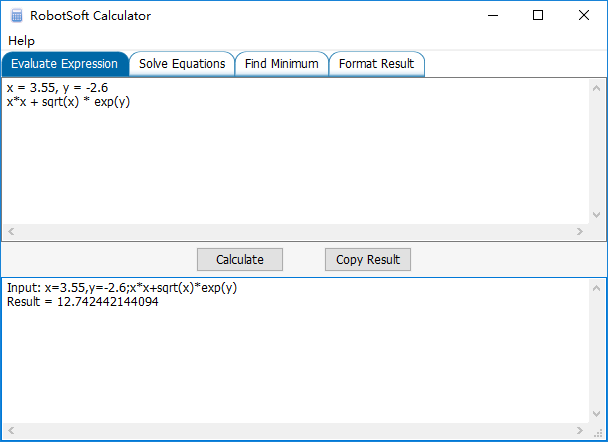You can enter multiple expressions at the same time.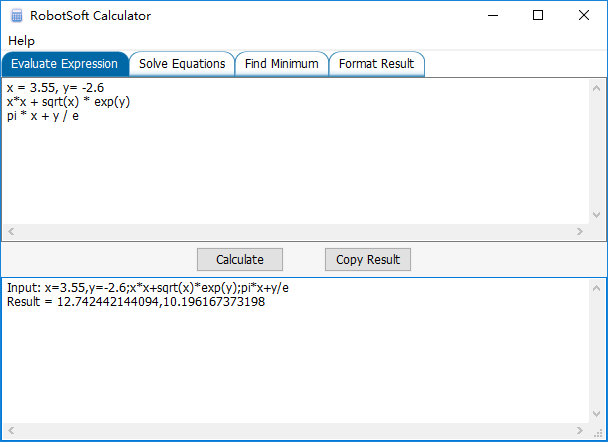Solve Equations Select the page "Solve Equations", then enter the equations which you want to solve, and then click the button "Solve".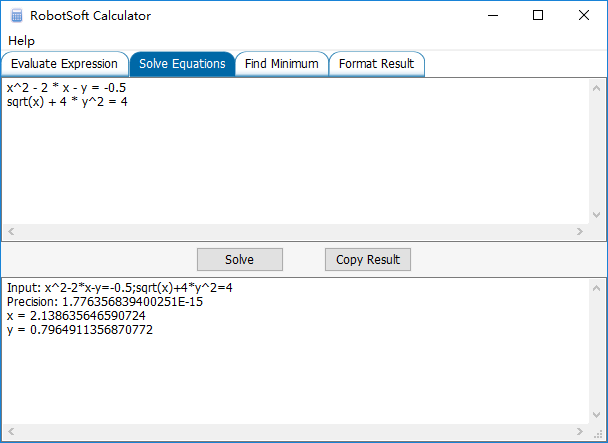The Solve Equations feature supports Greater Equal and Less Equal command.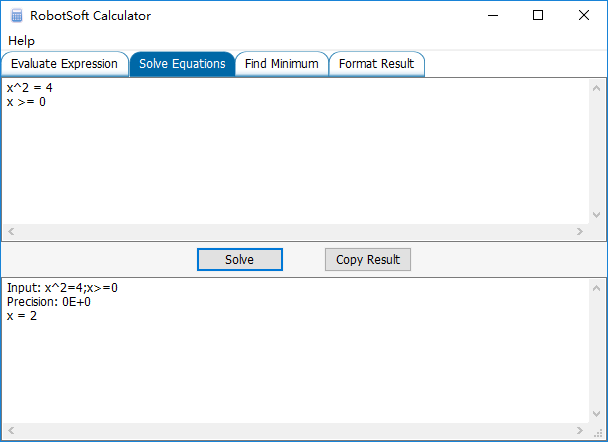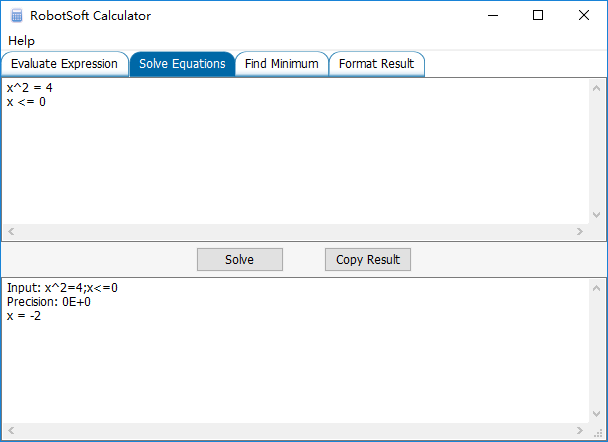Find Minimum Select the page "Find Minimum", then enter the function which you want to optimize, and then click the button "Find".http://www.robot-soft.com/   Copyright 2010-2016 by Robot-Soft.com Inc. All rights reserved.